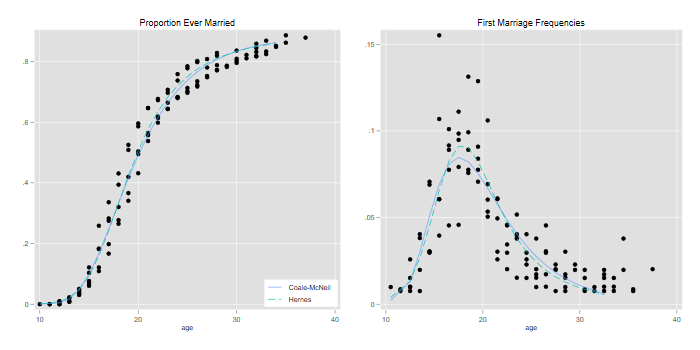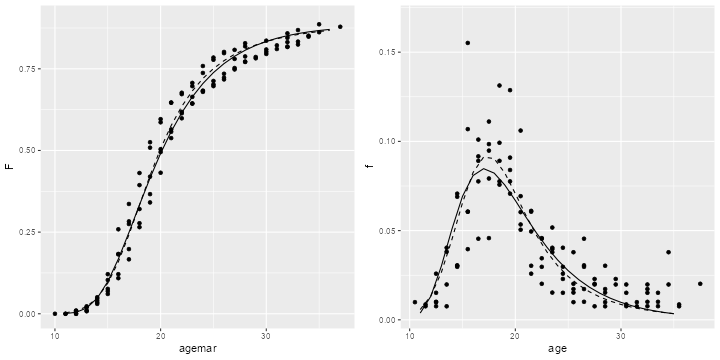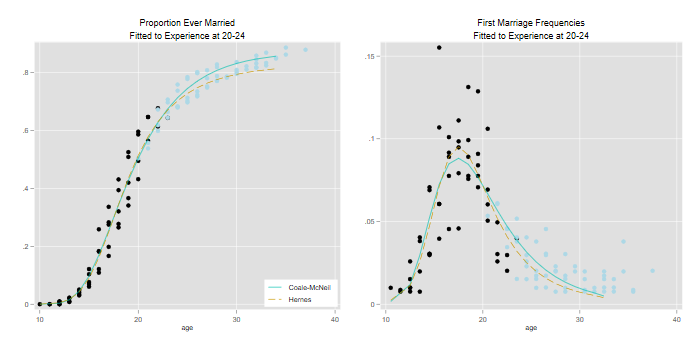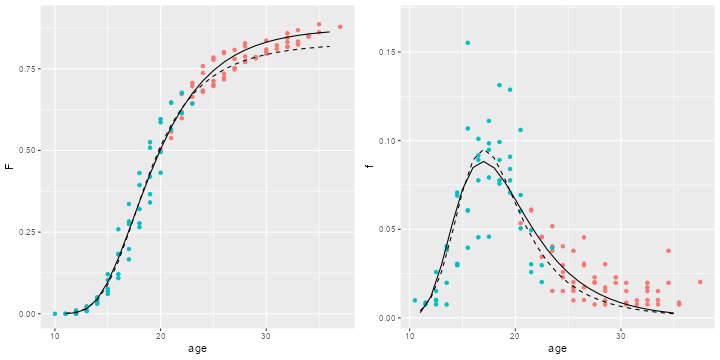Germán Rodríguez
Demographic Methods Princeton University## Nuptiality Models

We apply nuptiality models to age at marriage in Colombia using WFS data. The main objective is to show the use of models to extrapolate from incomplete cohort experience.

Note: the code below requires the command `nuptfer` in Stata, available here, just type `net from https://grodri.github.io/demography`. `nuptfer` package in R, which can be installed from GitHub.

### The Data

We read an extract with age at interview and age at marriage for the cohort 35-39 in the Colombia WFS with two variables, `v010` is age and `v109` is age at first union, coded missing if never in union. We treat women married at their current age as single at the start of the year, thereby avoiding assumptions about partial exposure, and then group the data by age and age at marriage

```. use https://grodri.github.io/datasets/co3539, clear
(COSR02 extract)

. replace v109 = . if v109 >= v010
(74 real changes made, 74 to missing)

. gen n = 1

. rename v010 age

. rename v109 agemar

. collapse (sum) n, by(age agemar)
```
```> library(haven)
> library(dplyr)
> cog <- mutate(co, v109 = ifelse(v109 >= v010, NA, v109)) |>
+   group_by(age = v010, agemar = v109) |> summarize( n = n() )
```

### Modeling the Complete Experience

We want to plot proportions ever married and first marriage frequencies for each of the single-year cohorts aged 35 to 39, together with fitted Coale-McNeil and Hernes models. We start by computing the observed frequencies

```. egen N = sum(n), by(age)

. gen f = n/N if !missing(agemar)
(5 missing values generated)

. bysort age (agemar): gen F = sum(f)-f
(5 missing values generated)
```
```> cog <- group_by(cog, age) |> mutate(f = n/sum(n), F = cumsum(f) - f)
```

Next we compute cumulative model schedules. The estimates for the Coale-McNeil model were obtained from the paper by Rodríguez and Trussell (1980), who report a mean of 20.4, a standard deviation of 5.4, and a proportion eventually marrying of 89%. The estimates for the Hernes model were computed using a similar maximum likelihood procedure, and yield an “attractiveness” parameter of 0.65 (at age 15), a decay rate of 0.15, and a proportion eventually marrying of 89%.

Given the parameters, the schedules temselves were computed using the `nuptfer` packages for Stata and R. The cumulative schedules were then differenced to obtain marriage frequencies by age.

```. egen CM = pnupt(agemar), mean(20.436) s(5.377) p(.885)
(5 missing values generated)
(5 missing values generated)
(5 missing values generated)
(5 missing values generated)

. quietly replace CM = . if age != 39 // use 39 to keep model

. egen H = phernes(agemar), a(0.6526) r(.1506) p(.8873)
(5 missing values generated)

. quietly replace H = . if age != 39 // use 39 to keep model

. sort age agemar // to be sure

. gen cm = (CM[_n+1] - CM)/(agem[_n+1] - agem)
(90 missing values generated)

. gen h = (H[_n+1] -H)/(agem[_n+1] - agem)
(90 missing values generated)
```
```> library(nuptfer)
> age <- seq(11, 36, 1)
> Fits <- data.frame(age = age,
+   Coale = pnupt(age, 20.436, 5.377, .885),
+   Hernes = phernes(age, 0.6526, 0.1506, 0.8873))
> dens <- function(x) c(diff(x),NA)
> fits <- mutate_each(Fits, funs(dens), -age)
```

We are ready to plot our results

```. twoway (scatter F agem )(line CM H agem, lp(solid dash)) ///
> , title(Proportion Ever Married) xtitle(age) name(F,replace) ///
>   legend(order(2 "Coale-McNeil" 3 "Hernes") ring(0) pos(5) col(1))

. gen agep = agem + 0.5
(5 missing values generated)

. twoway (scatter f agep )(line cm h agep, lp(solid dash)) ///
> , title(First Marriage Frequencies) xtitle(age) ///
>   legend(off) name(f,replace)

. graph combine F f, xsize(7) ysize(3.5)

. graph export co3539a.png, width(700) replace
file co3539a.png saved as PNG format
``````> library(ggplot2)
> library(gridExtra)
> g1 <- ggplot(cog, aes(agemar,F)) + geom_point() +
+   geom_line(data = Fits, aes(age, Coale), linetype=1) +
+   geom_line(data = Fits, aes(age, Hernes), linetype=2)
> g2 <- ggplot(cog, aes(agemar + 0.5, f)) + xlab("age") + geom_point() +
+   geom_line(data = fits, aes(age, Coale), linetype = 1) +
+   geom_line(data = fits, aes(age, Hernes), linetype = 2)
> g <- arrangeGrob(g1, g2, ncol = 2)
> ggsave("co3539ar.png", plot = g, width = 10, height = 5, dpi=72)
```The general fit of the models to noisy data is excellent. As usual, it is easier to appreciate differences working with densities rather than with cumulative distributions. The Hernes model leads to a distribution a bit more peaked than the Coale-McNeil (dashed and solid lines respectively).

### Predicting 15 Years Earlier

We will now simulate what would have happened if we had interviewed these cohorts 15 years earlier. We assume women who had married by then would have reported the same age at marriage, but the rest would have reported themselves as never married. To emphasize how little data we would have, I have redraw the plot below using a different color for data points “in the future”.

Rodrguez and Trussel (1980) fitted the Coale-McNeil to the censored cohort and obtained a mean of 20.2, a standard deviation of 5.1 and an estimated 87% eventually marrying. The fact that the estimates obtained when the cohort was 20-24 are so close to the estimates obtained when it was 35-39 is nothing short of remarkable.

I wemt ahead and fitted the Hernes model to the same censored experience and obtained an attractiveness parameter of 0.73 (at age 15), a rate of decay of 0.18 per year, and a proportion eventually marrying of 83%.

Here’s the code used for the two panels

```. // Left
. gen observed = agem < age-15

. egen CMc = pnupt(agem) , mean(20.1528) s(5.0955) p(.8740)
(5 missing values generated)
(5 missing values generated)
(5 missing values generated)
(5 missing values generated)

. quietly replace CMc = . if age != 39

. egen Hc = phernes(agem), a(0.7321) r(.1875) p(.8296)
(5 missing values generated)

. quietly replace Hc = . if age != 39

. twoway (scatter F agem if obs) (scatter F agem if !obs, color(ltblue)) ///
>   (line CMc Hc agem, lp(solid dash) ) , ///
>   title(Proportion Ever Married) subtitle(Fitted to Experience at 20-24) ///
>   legend(order(3 "Coale-McNeil" 4 "Hernes") ring(0) pos(5) col(1)) ///
>   xtitle(age) name(Fc,replace)

. // Right
. gen cmc = (CMc[_n+1]-CMc)/(agem[_n+1] - agem)
(90 missing values generated)

. gen hc = (Hc[_n+1] - Hc)/(agem[_n+1] - agem)
(90 missing values generated)

. twoway (scatter f agep if obs) (scatter f agep if !obs, color(ltblue)) ///
>   (line cmc hc agep, lp(solid dash)) ///
>   , title(First Marriage Frequencies) subtitle(Fitted to Experience at 20-24)
> ///
>     xtitle(age) legend(off) name(fc,replace)

. graph combine Fc fc, xsize(7) ysize(3.5)

. graph export co3539c.png, width(700) replace
file co3539c.png saved as PNG format
``````> cog <- mutate(cog,  observed = factor(agemar < age - 15, labels=c("no","yes")))
> Fits <- data.frame(age = age,
+   Coale = pnupt(age, 20.1528, 5.0955, 0.8740),
+   Hernes = phernes(age, 0.7321, 0.1875, 0.8296))
> fits <- mutate_each(Fits, funs(dens), -age)
> g1 <- ggplot(cog, aes(agemar,F, color=observed)) + xlab("age") + geom_point() +
+   geom_line(data = Fits, aes(age, Coale, color=NULL), linetype=1) +
+   geom_line(data = Fits, aes(age, Hernes, color=NULL), linetype=2) +
+   theme(legend.position = "none")
> g2 <- ggplot(cog, aes(agemar + 0.5, f, color=observed)) + xlab("age") + geom_point() +
+   geom_line(data = fits, aes(age, Coale, color=NULL), linetype=1) +
+   geom_line(data = fits, aes(age, Hernes, color=NULL), linetype=2) +
+   theme(legend.position = "none")
> g <- arrangeGrob(g1, g2, ncol = 2)
> ggsave("co3539cr.png", plot = g, width = 10, height = 5, dpi = 72)
```It seems clear that the Coale-McNeil does a better job predicting in this particular case. The Hernes model appears to adapt too well to the data at the younger ages, and as a result doesn’t predict as well for the older ages.

Things don’t always work this well. As shown in my paper with Trussell, the data for the oldest cohort, 45-49, are a bit more noisy and the prediction from the cohort’s early experience is not as good. Trying to predict the future course of nuptiality is a risky business, and all models rely on the basic assumption that the past says something about the future.

### Reference

Rodríguez G. and Trussell J. (1980). Maximum Likelihood Estimation of the Parameters of Coale’s Model Nuptiality Schedule from Survey Data. Word Fertility Survey Technical Bulletins*, No. 7. View on DHS site.

